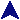Methods IIRuby Example Code# Place the array in a random order.  Floyd's alg.
def shuffle(arr)
for n in 0...arr.size
targ = n + rand(arr.size - n)
arr[n], arr[targ] = arr[targ], arr[n] if n != targ
end
end

# Make strange declarations.
def pairs(a, b)
a << 'Insane'
shuffle(b)
b.each { |x| shuffle(a); a.each { |y| print y, " ", x, ".\n" } }
end
first = ['Strange', 'Fresh', 'Alarming']